# Daily Coding Problem 1 - Revisited

You can find the original blog post here. The purpose of this post is to attempt a solution using a suggestion left by a reader, Josef Starychfojtu.

His suggestion was, and I quote:

This problem can be solved in O(n) with a hash map, (compared to yours O(n^2))

in which you hash the number in a way that if x + y = k, they would end up in the same slot.

Then you would just hash each element and check if the slot already contains some number, if yes, you found the pair.

As a recap, here is the original problem.

## Problem Statement

Given a list of numbers and a number `k`, return whether any two numbers from the list add up to `k`.

For example, given `[10, 15, 3, 7]` and `k` of 17, return true since 10 + 7 is 17.

Bonus: Can you do this in one pass?

## Solution

### Strategy 3 - F# Set

Let’s start by looking at what this solution will look like. We start with the formula provided by Josef, i.e. `x + y = k`.

Let’s assume that `x` is an element in the list and `k` is as defined in the Problem Statement.

In that case, we need to find out whether `y` is a member of the list. Or, put another way `{ y | y `` list, y = k - x }`.

In addition, we need to ensure that if `k = 2 * x`, which is to say that `x = y`, that there are two instances of `x` in the list.

So, how do we go about solving this problem?

1. Put the `x` elements (original elements of the list) into a set
2. For each `x`, find the `y` value using `y = k - x`
3. See if any `y` is in the set. If it is,
1. Check if `x = y`. If it does,
1. Ensure there are 2 `x` values in the list. If there are, return true. Else, return false.
2. Else return true.

NOTE: I am going to try once more to use BenchmarkDotNet to test this code.

``````// Easy way to work with any Nuget packages
Paket.Package
[ "FSharp.Collections.ParallelSeq"
"Expecto"
"Xplot.Plotly"
]

#r "/IfSharp/bin/packages/FSharp.Collections.ParallelSeq/lib/net45/FSharp.Collections.ParallelSeq.dll"
#r "/IfSharp/bin/packages/Expecto/lib/net461/Expecto.dll"
``````
``````// Open standard packages to assist with logic
open System
// Use Parallel operations to speed up execution
open FSharp.Collections.ParallelSeq

/// Quick debugging
let tee f x = f x |> ignore; x
``````

I will use the same function signature as the previous attempts, so that it is easy to benchmark them.

``````/// F# Set
let setRun ilist k =
/// Add all elements of ilist to a set
let xSet =
ilist
|> PSeq.fold (fun accum e -> Set.add e accum) Set.empty
H
/// Construct a list of y values.
let yList =
ilist
|> PSeq.map (fun x -> x, k - x)

/// Check if a value occurs at least twice in a list
let checkTwoOccurrences x lst =
lst
|> PSeq.filter ((=) x)
|> PSeq.length
|> (fun l -> l >= 2)

yList
|> PSeq.filter (fun (x, y) -> Set.contains y xSet)
|> PSeq.exists (fun (x, y) -> if x <> y then true else checkTwoOccurrences x ilist)
``````

Let’s test this function to make sure it works as expected, using the same tests as the original post.

``````open Expecto

/// Basic tests for the set to ensure the logic works correctly
let setTests =
testList "Set tests" [
test "[10; 15; 3; 7] 17" {
Expect.isTrue (setRun [10; 15; 3; 7] 17) "[10; 15; 3; 7] 17"
}

test "[10; 15; 3; 7] 18" {
Expect.isTrue (setRun [10; 15; 3; 7] 18) "[10; 15; 3; 7] 18"
}

test "[10; 15; 3; 7] 28" {
Expect.isFalse (setRun [10; 15; 3; 7] 28) "[10; 15; 3; 7] 28"
}

test "[10; 15; 3; 7] 20" {
Expect.isFalse (setRun [10; 15; 3; 7] 20) "[10; 15; 3; 7] 20"
}

test "[10; 15; 3; 7; 10] 20" {
Expect.isTrue (setRun [10; 15; 3; 7; 10] 20) "[10; 15; 3; 7; 10] 20"
}
]

runTests defaultConfig setTests
``````
``````    0
[13:56:47 INF] EXPECTO? Running tests... <Expecto>
[13:56:47 INF] EXPECTO! 5 tests run in 00:00:00.0311229 for Set tests – 5 passed, 0 ignored, 0 failed, 0 errored. Success! <Expecto>
``````

Our logic works. Let’s add one more strategy, because MSDN seems to imply that F#’s Set type / module is based on structural comparison (not that that’s an issue for integers).

### Strategy 4 - F# Map / Dictionary

We use the same strategy as #3, except using an F# `Map`.

``````/// F# Map
let mapRun ilist k =
/// Add all elements of ilist to a map
let xMap =
ilist
|> PSeq.map (fun x -> x, ())
|> Map.ofSeq

/// Construct a list of y values.
let yList =
ilist
|> PSeq.map (fun x -> x, k - x)

/// Check if a value occurs at least twice in a list
let checkTwoOccurrences x lst =
lst
|> PSeq.filter ((=) x)
|> PSeq.length
|> (fun l -> l >= 2)

yList
|> PSeq.filter (fun (x, y) -> Map.containsKey y xMap)
|> PSeq.exists (fun (x, y) -> if x <> y then true else checkTwoOccurrences x ilist)
``````

And, of course, a quick sanity check.

``````/// Basic tests for the map to ensure the logic works correctly
let mapTests =
testList "Map tests" [
test "[10; 15; 3; 7] 17" {
Expect.isTrue (mapRun [10; 15; 3; 7] 17) "[10; 15; 3; 7] 17"
}

test "[10; 15; 3; 7] 18" {
Expect.isTrue (mapRun [10; 15; 3; 7] 18) "[10; 15; 3; 7] 18"
}

test "[10; 15; 3; 7] 28" {
Expect.isFalse (mapRun [10; 15; 3; 7] 28) "[10; 15; 3; 7] 28"
}

test "[10; 15; 3; 7] 20" {
Expect.isFalse (mapRun [10; 15; 3; 7] 20) "[10; 15; 3; 7] 20"
}

test "[10; 15; 3; 7; 10] 20" {
Expect.isTrue (mapRun [10; 15; 3; 7; 10] 20) "[10; 15; 3; 7; 10] 20"
}
]

runTests defaultConfig mapTests
``````
``````    0
[13:56:47 INF] EXPECTO? Running tests... <Expecto>
[13:56:47 INF] EXPECTO! 5 tests run in 00:00:00.0305894 for Map tests – 5 passed, 0 ignored, 0 failed, 0 errored. Success! <Expecto>
``````

## Performance Comparison

Now that we have two new implementations which run in O(n), let’s perform some basic performance comparisons using BenchmarkDotNet.

I am importing the first two implementations from the first blog post.

``````/// Remove the first occurrence of an element from a list.  This is a safe method because
/// if the element does not occur, the list will be returned unchanged.
let removeFirstOccurrence e lst =
let rec loop accum = function
| [] -> List.rev accum
| h::t when e = h -> (List.rev accum) @ t
| h::t -> loop (h::accum) t
loop [] lst

/// Brute force method
let bruteForceRun ilist k =
ilist
|> PSeq.map (fun e -> e, removeFirstOccurrence e ilist)
|> PSeq.collect (fun (e,remElem) -> PSeq.map (fun re -> re + e) remElem)
|> PSeq.exists (( = ) k)

/// Subtraction method
let subtractionRun ilist k =
ilist
|> PSeq.map (fun e -> (k - e), removeFirstOccurrence e ilist)
|> PSeq.exists (fun (rem, remL) -> PSeq.exists ((=) rem) remL)
``````

As well as the input generator.

``````/// Performance testing setup - change the input parameter if you want repeatable tests
/// NOTE: System.Random is NOT thread-safe.  Using PSeq here is dubious at best.
let random = Random((int) DateTime.Now.Ticks &&& 0x0000FFFF)
let mutable randLock = Object()

/// Convenience method to generate random numbers safely.
let getNextRand (min, max) = lock randLock (fun () -> random.Next (min, max))

/// Represents inputs to the algorithms, with type (int list, int) list.
let inputGenerator numToGenerate : (int list * int) list =
// Generate a single input
let generateOne () =
// list length is between 10 and 10,000 entries
let listLen = getNextRand (10, 10000)

// limiting each list element to be between 0 and 1,000,000
let lst =
PSeq.init listLen
(fun _ -> getNextRand (0, 1000000))
|> PSeq.toList

// k can go up to 2,000,000, which is double the maximum entry in the list
let k = getNextRand (0, 2000000)

lst, k

PSeq.init numToGenerate (fun _ -> generateOne())
|> PSeq.toList
``````

Despite various attempts, BenchmarkDotNet refuses to run within jupyter :(. So, instead, measuring performance using the `Stopwatch` class and `System.Diagnostics`.

``````/// Control variable for run: how many entries in each run?
let numToGenerate = 150

/// Control variable for run: how many runs?
let numberOfRuns = 10

/// Convenience function to run a performance test against a set of inputs.
let performanceTest runner inputs =
[ for i in 0 .. List.length inputs - 1 do
yield runner (fst inputs.[i]) (snd inputs.[i]) ]
|> ignore

/// Each run has different inputs
let inputsForRuns =
[ for i in 0 .. numberOfRuns-1 do
yield inputGenerator numToGenerate ]

/// Force-run the garbage collector
let cleanup () =
GC.Collect()
GC.WaitForPendingFinalizers()
GC.Collect()

/// Measure performance indicators from System.Diagnostics
let measureDiagnostics () = [
System.Diagnostics.Process.GetCurrentProcess().UserProcessorTime.TotalMilliseconds
System.Diagnostics.Process.GetCurrentProcess().PrivilegedProcessorTime.TotalMilliseconds
System.Diagnostics.Process.GetCurrentProcess().TotalProcessorTime.TotalMilliseconds
(System.Diagnostics.Process.GetCurrentProcess().VirtualMemorySize64 |> float) / 1048576.
(System.Diagnostics.Process.GetCurrentProcess().WorkingSet64 |> float) / 1048576.
(System.Diagnostics.Process.GetCurrentProcess().PrivateMemorySize64 |> float) / 1048576.
]

/// Diff two outputs from `measureDiagnostics`
let diff (lafter:float list) (lbefore:float list) =
List.zip lafter lbefore
|> List.map (fun (a, b) -> a - b)

/// Stopwatch to measure runtime performance.
let stopwatch = System.Diagnostics.Stopwatch()

/// Capture runtimes and memory usage.
let mutable runtimeAndMemory : float list list = []

// Separate the runs between cells to avoid a timeout on a single cell.
``````
``````// Sleeping to ensure GC cleanup is done before next cell runs
cleanup ()
``````
``````// Brute Force Run
stopwatch.Reset()
let beforeBFR = measureDiagnostics ()
stopwatch.Start()

for inputs in inputsForRuns do
performanceTest bruteForceRun inputs

stopwatch.Stop()
let afterBFR = measureDiagnostics ()
runtimeAndMemory <- runtimeAndMemory @
[stopwatch.Elapsed.TotalSeconds::(diff afterBFR beforeBFR)]
``````
``````// Sleeping to ensure GC cleanup is done before next cell runs
cleanup ()
``````
``````// Subtraction Run
stopwatch.Reset()
let beforeSR = measureDiagnostics ()
stopwatch.Start()

for inputs in inputsForRuns do
()
performanceTest subtractionRun inputs

stopwatch.Stop()
let afterSR = measureDiagnostics ()
runtimeAndMemory <- runtimeAndMemory @
[stopwatch.Elapsed.TotalSeconds::(diff afterSR beforeSR)]
``````
``````// Sleeping to ensure GC cleanup is done before next cell runs
cleanup ()
``````
``````// Set Run
stopwatch.Reset()
let beforeSeR = measureDiagnostics ()
stopwatch.Start()

for inputs in inputsForRuns do
()
performanceTest setRun inputs

stopwatch.Stop()
let afterSeR = measureDiagnostics ()
runtimeAndMemory <- runtimeAndMemory @
[stopwatch.Elapsed.TotalSeconds::(diff afterSeR beforeSeR)]
``````
``````// Sleeping to ensure GC cleanup is done before next cell runs
cleanup ()
``````
``````// Map Run
stopwatch.Reset()
let beforeMR = measureDiagnostics ()
stopwatch.Start()

for inputs in inputsForRuns do
()
performanceTest mapRun inputs

stopwatch.Stop()
let afterMR = measureDiagnostics ()
runtimeAndMemory <- runtimeAndMemory @
[stopwatch.Elapsed.TotalSeconds::(diff afterMR beforeMR)]
``````

### Plot the performance results

And now we can visualize the results. We start with the raw numbers.

``````open XPlot.GoogleCharts

let rows = [
"SW-s"
"UserCPU-s"
"PrivCPU-s"
"TotCPU-s"
"VirtMem-MB"
"WorkSet-MB"
"PrivMem-MB"
]

let corner = "Measurement / Algs"

let columns = ["Brute Force";"Subtraction";"Set";"Map"]
``````
``````[ for i in runtimeAndMemory do yield List.zip rows i ]
|> Chart.Table
|> Chart.WithOptions (Options(showRowNumber=true))
|> Chart.WithLabels (corner :: columns)
``````
Measurement / Algs Brute Force Subtraction Set Map
1 SW-s 778.217 512.085 8.016 9.924
2 UserCPU-s 1,137,120 676,610 7,840 10,710
3 PrivCPU-s 154,730 122,920 810 920
4 TotCPU-s 1,291,850 799,530 8,650 11,630
5 VirtMem-MB 50.602 160.57 0 -179.926
6 WorkSet-MB 28.867 158.93 0.363 -179.348
7 PrivMem-MB 50.617 160.57 0 -179.926

And we can see the percentage increase or decrease compared to Brute Force (arbitrarily chosen as the baseline). Note that the Brute Force column shows 0% it is the baseline.

``````/// Function to transpose a 2D list.
let rec transpose = function
| [] -> failwith "cannot transpose a 0-by-n matrix"
| []::xs -> []
| xs -> List.map List.head xs :: transpose (List.map List.tail xs)

/// A reference to the transposed results.
let transposedRAM = transpose runtimeAndMemory

/// Separate chart to conver the raw numbers into percentages.
let percentagesRAM =
[ for measure in transposedRAM do
let baseline = List.item 0 measure
yield
measure
|> List.map (fun elem -> 100. * (elem - baseline) / baseline)
]
|> transpose

[ for i in percentagesRAM do yield List.zip rows i ]
|> Chart.Table
|> Chart.WithOptions (Options(showRowNumber=true, allowHtml=true))
|> Chart.WithLabels (corner :: columns)
``````
Measurement / Algs Brute Force Subtraction Set Map
1 SW-s 0 -34.198 -98.97 -98.725
2 UserCPU-s 0 -40.498 -99.311 -99.058
3 PrivCPU-s 0 -20.558 -99.477 -99.405
4 TotCPU-s 0 -38.11 -99.33 -99.1
5 VirtMem-MB 0 217.323 -100 -455.574
6 WorkSet-MB 0 450.555 -98.742 -721.286
7 PrivMem-MB 0 217.225 -100 -455.464

And a column chart.

``````[ for i in runtimeAndMemory do yield List.zip rows i ]
|> Chart.Column
|> Chart.WithOptions (Options(height=750))
|> Chart.WithLabels (corner :: columns)
``````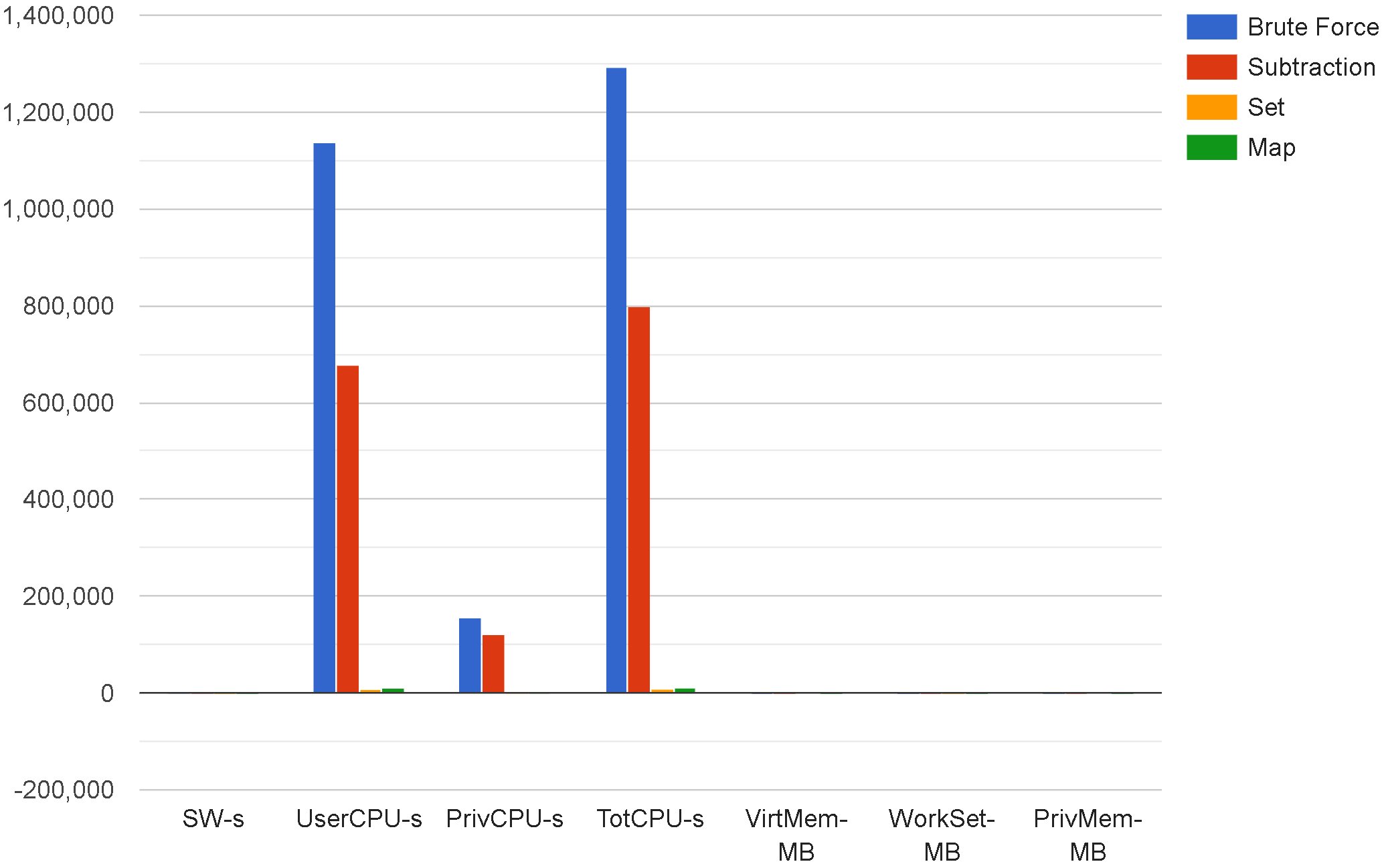### Individual charts

This section contains individual charts, one for each of the 7 data points in the table / column chart above.

``````List.zip columns (List.item 0 transposedRAM)
|> Chart.Bar
|> Chart.WithOptions(Options(legendPosition="top"))
|> Chart.WithLabel (List.item 0 rows)
``````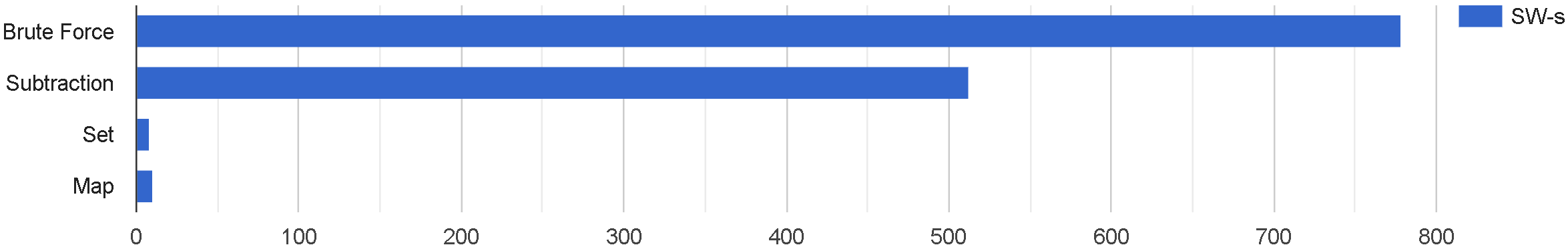``````List.zip columns (List.item 1 transposedRAM)
|> Chart.Bar
|> Chart.WithOptions(Options(legendPosition="top"))
|> Chart.WithLabel (List.item 1 rows)
``````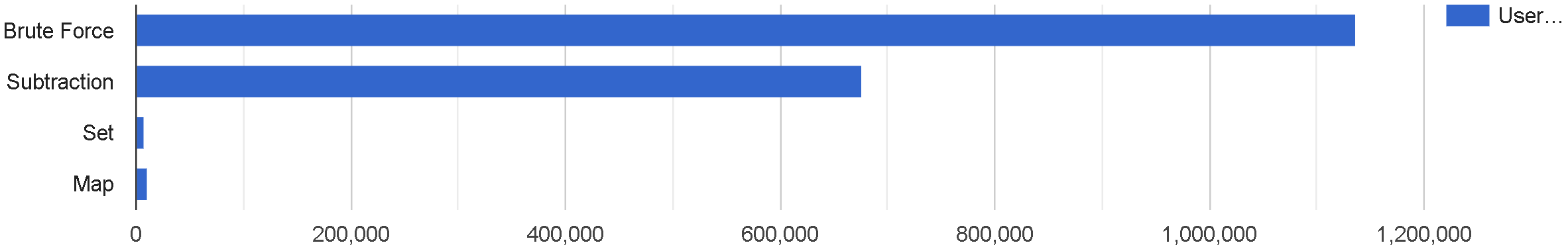``````List.zip columns (List.item 2 transposedRAM)
|> Chart.Bar
|> Chart.WithOptions(Options(legendPosition="top"))
|> Chart.WithLabel (List.item 2 rows)
``````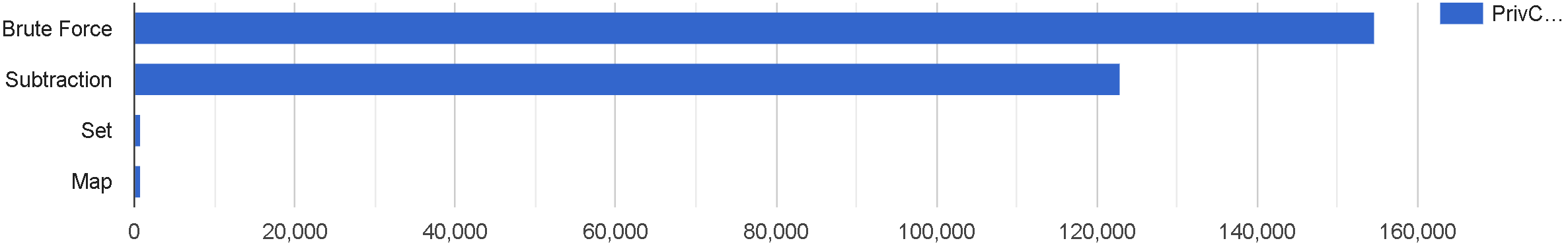``````List.zip columns (List.item 4 transposedRAM)
|> Chart.Bar
|> Chart.WithOptions(Options(legendPosition="top"))
|> Chart.WithLabel (List.item 4 rows)
``````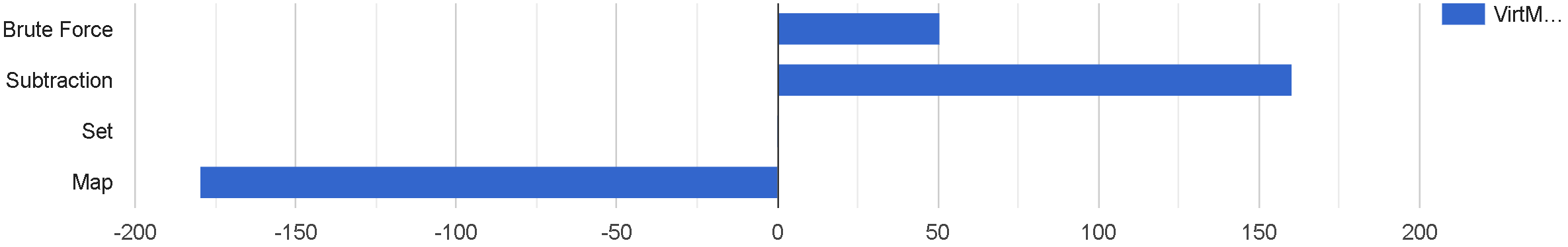``````List.zip columns (List.item 5 transposedRAM)
|> Chart.Bar
|> Chart.WithOptions(Options(legendPosition="top"))
|> Chart.WithLabel (List.item 5 rows)
``````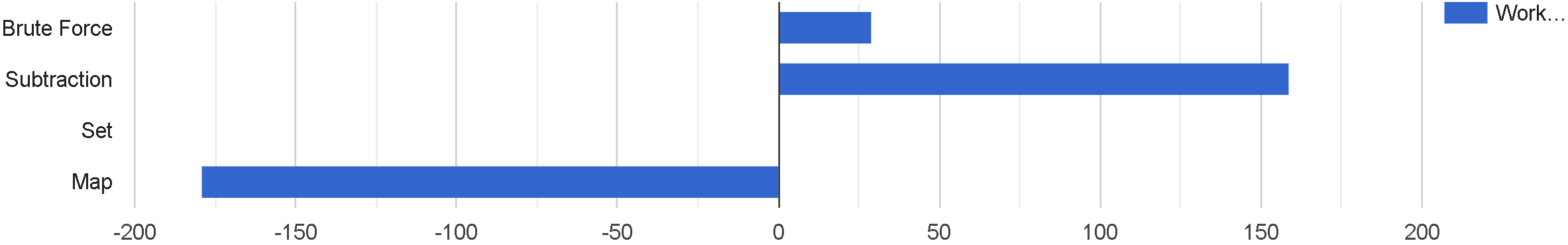``````List.zip columns (List.item 6 transposedRAM)
|> Chart.Bar
|> Chart.WithOptions(Options(legendPosition="top"))
|> Chart.WithLabel (List.item 6 rows)
``````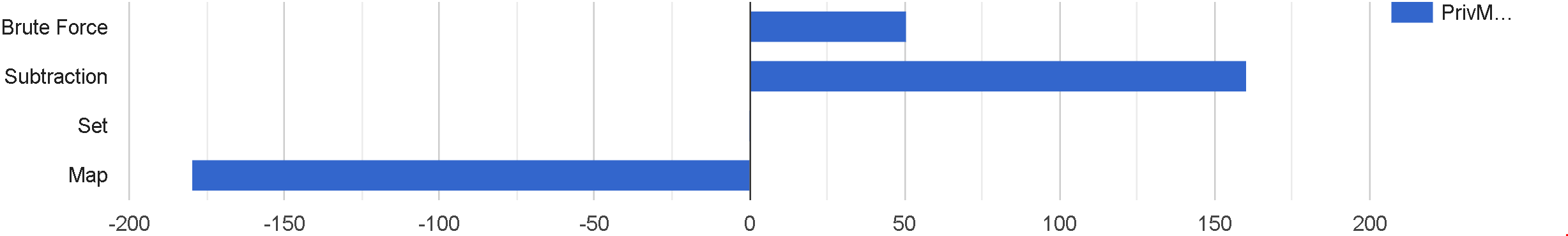## Conclusion

The results are quite definitive, assuming the data can be considered reliable (i.e. tracking Memory and CPU usage from within IFSharp/Jupyter, running on Mono).

The Subtraction method is still ~36% faster than Brute Force. However, with the Set and Map methods, there was an over-98% decrease in run-time and 98-99% decrease in CPU usage.

Measuring memory usage, on the other hand, is a complete mess. Every time I ran this Jupyter notebook, the system recorded wildly different reading for memory, often showing negative values. I have left the latest readings available above, but please take them with a large grain of salt.

Regardless of the memory situation, this problem clearly shows that algorithmic complexity and selecting the appropriate data structure can have a huge impact on your system’s performance. Going from `O(n^2)` to `O(n)` (or better) had a drastic impact on processing time and CPU usage.

Once again, I’d like to thank Josef for the suggestion, because it provided an opportunity to see some very interesting results. See you in the next one!